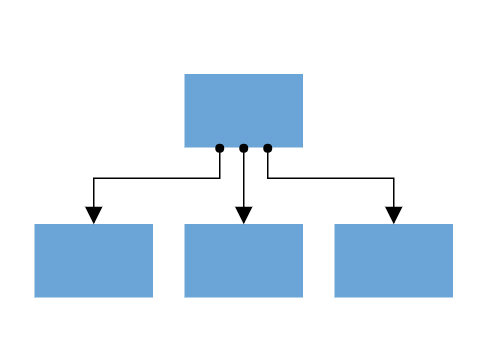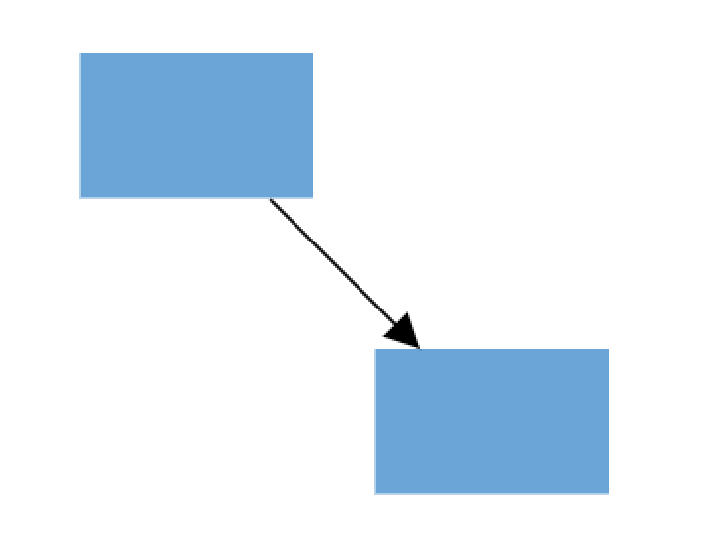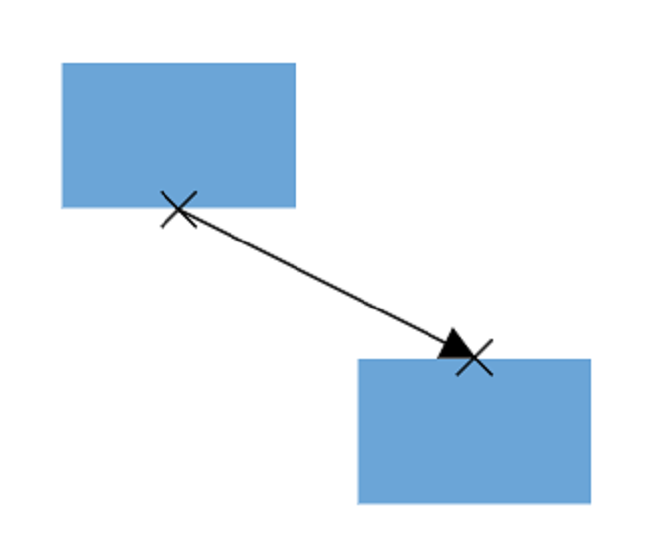Search results

# Port in React Diagram component

24 Jun 2022 / 7 minutes to read

Diagram provides support to define custom ports for making connections.When a connector is connected between two nodes, its end points are automatically docked to the node’s nearest boundary as shown in the following image.Ports act as the connection points of the node and allows to create connections with only those specific points as shown in the following image.## Add ports when initializing nodes

To add a connection port, define the port object and add it to node’s ports collection. The `offset` property of port accepts an object of fractions and used to determine the position of ports. The following code illustrates how to add ports when initializing the node.

``````import * as React from "react";
import * as ReactDOM from "react-dom";
import {
Diagram,
DiagramComponent,
NodeModel,
PortVisibility
} from "@syncfusion/ej2-react-diagrams";

// A node is created and stored in nodes array.

let node: NodeModel[] = [{
// Position of the node
offsetX: 250,
offsetY: 250,
// Size of the node
width: 100,
height: 100,
style: {
fill: '#6BA5D7',
strokeColor: 'white'
},
// Initialize port collection
ports: [{
// Sets the position for the port
offset: {
x: 0.5,
y: 0.5
},
visibility: PortVisibility.Visible
}]
}];

// initialize Diagram component

ReactDOM.render( <
DiagramComponent id = "diagram"
width = {
'100%'
}
height = {
'600px'
}
nodes = {
node
}
// render initialized Diagram
>
< /DiagramComponent>, document.getElementById("diagram") );``````

Add ports at runtime by using the client-side method `addPorts`. The following code illustrates how to add ports to node at runtime.

The port’s ID property is used to define the unique ID for the port and its further used to find the port at runtime. If ID is not set, then default ID is automatically set.

``````import * as React from "react";
import * as ReactDOM from "react-dom";
import {
Diagram,
DiagramComponent,
NodeModel,
PointPortModel,
PortVisibility
} from "@syncfusion/ej2-react-diagrams";

let diagramInstance:DiagramComponent;

// A node is created and stored in nodes array.
let node: NodeModel[] = [{
// Position of the node
offsetX: 250,
offsetY: 250,
// Size of the node
width: 100,
height: 100,
style: {
fill: '#6BA5D7',
strokeColor: 'white'
},
}];
// Initialize port collection
let port: PointPortModel[] = [{
id: 'port1',
offset: {
x: 0,
y: 0.5
},
visibility: PortVisibility.Visible
} {
id: 'port2',
offset: {
x: 1,
y: 0.5
},
visibility: PortVisibility.Visible
},
{
id: 'port3',
offset: {
x: 0.5,
y: 0
},
visibility: PortVisibility.Visible
},
{
id: 'port4',
offset: {
x: 0.5,
y: 1
},
visibility: PortVisibility.Visible
}
];

// initialize Diagram component
ReactDOM.render( < DiagramComponent id = "diagram"  ref={diagram => diagramInstance = diagram}
width = {
'100%'
}
height = {
'600px'
}
nodes = {
node
}
// render initialized Diagram
/>,   document.getElementById("diagram") );
// Method to add ports through run time

## Remove ports at runtime

Remove ports at runtime by using client-side method `removePorts`. Refer to the following example which shows how to remove ports at runtime.

Source
Preview
index.jsx
index.tsx
``````import * as React from "react";
import * as ReactDOM from "react-dom";
import { DiagramComponent, PortVisibility } from "@syncfusion/ej2-react-diagrams";
// A node is created and stored in nodes array.
let node = [{
// Position of the node
offsetX: 250,
offsetY: 250,
// Size of the node
width: 100,
height: 100,
style: {
fill: '#6BA5D7',
strokeColor: 'white'
},
// Initialize port collection
ports: [{
id: 'port1',
offset: {
x: 0,
y: 0.5
},
visibility: PortVisibility.Visible
},
{
id: 'port2',
offset: {
x: 1,
y: 0.5
},
visibility: PortVisibility.Visible
},
{
id: 'port3',
offset: {
x: 0.5,
y: 0
},
visibility: PortVisibility.Visible
},
{
id: 'port4',
offset: {
x: 0.5,
y: 1
},
visibility: PortVisibility.Visible
}
]
}];
let diagramInstance;
// initialize Diagram component
let ports = [{
id: 'port1',
}, {
id: 'port2',
}, {
id: 'port3',
}, {
id: 'port4',
}];
ReactDOM.render(<DiagramComponent id="diagram" ref={diagram => (diagramInstance = diagram)} width={'100%'} height={'600px'} nodes={node} created={() => {
diagramInstance.removePorts(diagramInstance.nodes, ports);
}}/>, document.getElementById("diagram"));``````
``````import * as React from "react";
import * as ReactDOM from "react-dom";
import {
Diagram,
DiagramComponent,
NodeModel,
Node,
PointPortModel,
PortVisibility
} from "@syncfusion/ej2-react-diagrams";

// A node is created and stored in nodes array.

let node: NodeModel[] = [{
// Position of the node
offsetX: 250,
offsetY: 250,
// Size of the node
width: 100,
height: 100,
style: {
fill: '#6BA5D7',
strokeColor: 'white'
},
// Initialize port collection
ports: [{
id: 'port1',
offset: {
x: 0,
y: 0.5
},
visibility: PortVisibility.Visible
},
{
id: 'port2',
offset: {
x: 1,
y: 0.5
},
visibility: PortVisibility.Visible
},
{
id: 'port3',
offset: {
x: 0.5,
y: 0
},
visibility: PortVisibility.Visible
},
{
id: 'port4',
offset: {
x: 0.5,
y: 1
},
visibility: PortVisibility.Visible
}
]
}];
let diagramInstance:DiagramComponent;
// initialize Diagram component
let ports: PointPortModel[] = [{
id: 'port1',
}, {
id: 'port2',
}, {
id: 'port3',
}, {
id: 'port4',
}];
ReactDOM.render( <DiagramComponent id = "diagram"  ref={diagram => (diagramInstance = diagram)}
width = {
'100%'
}
height = {
'600px'
}
nodes = {
node
}
created = {
() => {
diagramInstance.removePorts(diagramInstance.nodes, ports);
}
}
// render initialized Diagram
/>,   document.getElementById("diagram") );``````

## Update port at runtime

You can change any port properties at runtime and update it through the client-side method `dataBind`.

The following code example illustrates how to change the port properties.

``````import * as React from "react";
import * as ReactDOM from "react-dom";
import {
Diagram,
DiagramComponent,
NodeModel,
PortVisibility
} from "@syncfusion/ej2-react-diagrams";

// A node is created and stored in nodes array.

let node: NodeModel[] = [{
// Position of the node
offsetX: 250,
offsetY: 250,
// Size of the node
width: 100,
height: 100,
style: {
fill: '#6BA5D7',
strokeColor: 'white'
},
// Initialize port collection
ports: [{
offset: {
x: 0.5,
y: 0.5
},
visibility: PortVisibility.Visible
}]
}];
let diagramInstance:DiagramComponent;
// initialize Diagram component

ReactDOM.render( < DiagramComponent id = "diagram"  ref={diagram => diagramInstance = diagram}
width = {
'100%'
}
height = {
'600px'
}
nodes = {
node
}
// render initialized Diagram
/>,   document.getElementById("diagram") );
diagramInstance.nodes.ports.offset = {
x: 1,
y: 1
};
diagramInstance.dataBind();``````

## Appearance

The following code illustrates how to change the appearance of port.

Source
Preview
index.jsx
index.tsx
``````import * as React from "react";
import * as ReactDOM from "react-dom";
import { DiagramComponent, PortVisibility } from "@syncfusion/ej2-react-diagrams";
// A node is created and stored in nodes array.
let node = [{
// Position of the node
offsetX: 250,
offsetY: 250,
// Size of the node
width: 100,
height: 100,
style: {
fill: '#6BA5D7',
strokeColor: 'white'
},
// Initialize port collection
ports: [{
offset: {
x: 1,
y: 0.5
},
visibility: PortVisibility.Visible,
//Set the style for the port
style: {
fill: 'red',
strokeWidth: 2,
strokeColor: 'black'
},
width: 12,
height: 12,
// Sets the shape of the port as Circle
shape: 'Circle'
}]
}];
let diagramInstance;
// initialize Diagram component
ReactDOM.render(<DiagramComponent id="diagram" ref={diagram => diagramInstance = diagram} width={'100%'} height={'600px'}
nodes={node}/>, document.getElementById("diagram"));``````
``````import * as React from "react";
import * as ReactDOM from "react-dom";
import {
Diagram,
DiagramComponent,
NodeModel,
PortVisibility
} from "@syncfusion/ej2-react-diagrams";

// A node is created and stored in nodes array.

let node: NodeModel[] = [{
// Position of the node
offsetX: 250,
offsetY: 250,
// Size of the node
width: 100,
height: 100,
style: {
fill: '#6BA5D7',
strokeColor: 'white'
},
// Initialize port collection
ports: [{
offset: {
x: 1,
y: 0.5
},
visibility: PortVisibility.Visible,
//Set the style for the port
style: {
fill: 'red',
strokeWidth: 2,
strokeColor: 'black'
},
width: 12,
height: 12,
// Sets the shape of the port as Circle
shape: 'Circle'
}]
}];
let diagramInstance: DiagramComponent;
// initialize Diagram component

ReactDOM.render( < DiagramComponent id = "diagram"  ref={diagram => diagramInstance = diagram}
width = {
'100%'
}
height = {
'600px'
}
The constraints property allows to enable/disable certain behaviors of ports. For more information about port constraints, refer to `Port Constraints`.